# Electric Energy

## Electricity of Class 10

To maintain an electric current to pass through the conductor, a continuous work has to be done. The total work done by a current in an electric circuit is known as Electric Energy.

## DERIVATION OF WORK DONE AND ELECTRIC ENERGY

Now we know that:

Current (I) = charge(Q)/ time(t)  or Q = I T ... (i)

Also, potential difference is the work done in bringing a charge Q from one end to the other end of the conductor. Thus

(Potential Difference) V = W/Q

W = VQ ... (ii)

From (i) and (ii), we get that

W = V(It)

W = VIT ... (iii)

But according to Ohm's law

V = IR ... (iv)

From equation (iii) and (iv), we get that

W = (IR)(IT)

W = I2 Rt

But I = V/R and by putting this value in equation (iii), we get

W =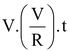W =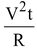This work done (W) by the current, measures the Electric Energy. Thus

W ∝ I2

W ∝ R

W ∝ t

Thus,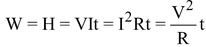(i) Commercial unit of electrical energy (Kilo watt - hour):

The S.I. unit of electrical energy is Joule and we know that for commercial purposes we use a bigger unit of electrical energy which is called "Kilowatt - hour". One Kilowatt - hour is the amount of electri-cal energy consumed when an electrical appliance having a power rating of 1 Kilowatt and is used for 1 Hour.

### (ii) Relation between Kilowatt hour and Joule:

Kilowatt-hour is the energy supplied by a rate of working of 1000 watts for 1 hour.

1 kilowatt-hour= 3600000 joules

⇒ 1kWh=3.6 × 106J

### ELECTRIC POWER:

The rate at which electric energy is dissipated or consumed, is termed as electric power. The power P is given by, P = W/t = I2 R

The unit of electric power is watt, which is the power consumed when 1 A of current flows at a potential difference of 1V.

### Unit of power:

The S.i. unit of electric power is ‘watt' which is denoted by the letter W. The power of 1 watt is a rate of working of 1 joule per second.

A bigger unit of electric power is kilowatt.

1 kilowatt (kW) = 1000 watt.

Power of an agent is also expressed in horse power (hp).

1 hp = 746 watt.

(ii) Formula for calculating electric power:

We know, Power, P = Work/Time

And Work, W = V × I × t Joules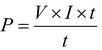P = V × I

Power P in terms of I and R :Now from Ohm's law we have, V/I = R

V= I × R

P= I × R × I

P= I2 × R

Power P in terms of V and R: We know, P = V × I

From Ohm's law I = V/R

P = V x V/R

P = V2/R

### Power - Voltage Rating of Electrical Appliances:

Every electrical appliance like an electric bulb, radio or fan has a label or engraved plate on it which tells us the voltage (to be applied) and the electrical power consumed by it. For example, if we look at a particular bulb in our home, it may have the figures 220V, 100W written on it. Now, 220V means that this bulb is to be used on a voltage of 220 volts and 100W which means it has a power consumption of 100 watts or 100 joules per second.

### ELECTRIC FUSE:

An electric fuse is an easily fusible wire of short length put into an electrical circuit for protection purposes. It is arranged to melt ("blow") at a definite current. It is an alloy of lead and tin (37% lead + 63% tin). It has a low resistivity and low melting point. As soon as the safe limit of current exceeds, the fuse "blows" and the electric circuit is cut off.

Consider a wire of length L, radius r and resistivity ρ . Let I be the current flowing through the wire. Now rate at which heat is produced in the wire is,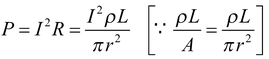This heat increases the temperature of the wire. Due to radiation some heat is lost. The temperature of the fuse becomes constant when the heat lost due to the radiation becomes equal to the heat produced due to the passage of current. This gives the value of current which can safely pass through the fuse.

In other words we have,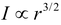1. 15 bulbs of 60W each, run for 6 hours daily and a refrigerator of 300 W runs for 5 hours daily. Work out per day bill at 3 rupees per unit.

Solution: Total wattage of 15 bulbs = 15 × 60W = 900W

∴ Electrical energy consumed by bulbs per day = P × t = 900 × 6 = 5400Wh

And electrical energy consumed by refrigerator per day = 300 × 5 = 1500 h

Total electrical energy consumed per day = (5400 + 1500)Wh = 6900Wh

∴ Electrical energy consumed per day = 6900/1000 KWh = 6.9 KWh

Here, per day bill = Rs. 6.9 × 3 = Rs. 20.7

2. Two lamps, one rated 100W at 220V and other 60W at 220V are connected in parallel to a 220V supply. What is current drawn from the supply line?

Solution: Given that V = 220V

P1 = 100W and P2 = 60W

∴ Current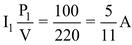Similarly,

Current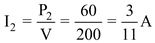Hence, total current drawn from the supply line =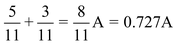.

### HOUSE-HOLD ELECTRICAL CIRCUIT:

Electric power is usually generated at places which are very far from the places where it is consumed. At the generating station, the electric power is generated at 11,000 volt (because voltage higher than this causes insulation difficulties, while the voltage lower than this involves high current). This voltage is alternating of frequency 50 Hz (i.e. changing its polarity 50 times in a second). The power is transmitted over long distances at high voltage to minimise the loss of energy in the transmission line wires. For a given electric power, the current becomes low at a high voltage and therefore the loss of energy due to heating (=I2 Rt) becomes less. Thus, the alternating voltage is stepped up from 11 kV to 132 kV at the generating station (or called grid sub-station). It is then transmitted to the main sub-station. At the main sub-station, this voltage is stepped down to 33 kV and is transmitted to the switching transformer station or the city sub-station. At the city sub-station, it is further stepped down to 220 V for supply to the consumer as shown in figure.

To supply power to a house either the overhead wires on poles are used or an underground cable is used. Before the electric line is connected to the meter in a house, a fuse of high rating (≈ 50 A) is connected at the pole or before the meter. This is called the company fuse. The cable used for connection has three wires: (i) live (or phase) wire, (ii) neutral wire and (iii) earth wire. The neutral and the earth wires are connected together at the local sub-station, so the neutral wire is at the earth potential. After the company fuse, the cable is connected to a kWh meter. From the meter, connections are made to the distribution board through a main fuse and a main switch.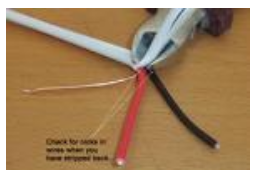The main switch is a double pole switch. It has iron covering. The covering is earthed. This switch is used to cut the connections of the live as well as the neutral wires simultaneously. The main switch and the meter are locally earthed (in the compound of house). From the distribution board, the wires go to the different parts of the house.

There are two systems of wiring which are in common use:

• the tree system
• the ring system

### Tree System:

In this system, different branch lines are taken from the distribution board for the different parts of the house. These branch lines look like the different branches of a tree. Each branch line is taken to a room through a fuse in the live wire. The different circuits are connected in parallel so that if there is a short circuiting in one distribution circuit, its fuse will blow off, without affecting the electric supply in the other circuits. The neutral N and the earth E are common for all circuits. The connection to the neutral N is to complete the circuit. All the appliances in a room are connected in parallel so that they work at the same voltage. The line wires used for connections should be of proper current carrying capacity depending on the rating of the appliance to avoid their overheating. The overheating in line often results in fire. The switches and sockets should also have the proper current carrying capacity.

•  It requires plugs and sockets of different sizes for different current carrying capacities.
•  When the fuse in one distribution line blows, it disconnetcts all the appliances in the distributin line.
• This wiring is expensive.
•  If a new appliance is to be installed requiring higher current, say 15A, while the original circuit in the room is for 5A rating, then it is necessary to put new leads upto the distribution box. This could be quite expensive and inconvenient.

### Ring System:

The ring-system of electric wiring is now rapidly replacing the older tree system described above. It consists of a ring-circuit. Wires starting from the main fuse-box, run around all the main rooms of the house and then come back to the fuse-box again. The fuse box contains a fuse of rating about 30 A. A separate connection is taken from the live wire of the ring for each appliance. The terminal of the appliance is connected to the live wire through a separate fuse and a switch. If the fuse of one appliance burns, it does not affect the other apliances. For each appliance, the wires used for connection should be of proper current carrying capacity.

It can be noted that the current can travel to an individual appliance through two separate paths. Thus effectively the connection for each appliance is through double thickness of wire. Therefore the wire used for ring main is of a lower rating than that which would be required for a direct connection to the mains. This reduces the cost of wiring considerably. Plugs and sockets of the same size can be used, but each plug should have its own fuse of rating suitable for the particular appliance.

Another advantage of this system is in installing a new appliance, since a new line up to the distribution box is not required. The appliance can be directly connected to ring main in the room. The only consideration is that the total load on the ring circuit should not exceed the main fuse viz. 30 A.

### Domestic Heating Applications:

Electric appliances like iron, heater, radiator etc. depend on the fact that when a current is sent through a wire, the wire is heated up and it begins to radiate energy.

The most widely used material for making the heater wire is nichrome. It is an alloy of nickel and chromium in the ratio of 4 : 1. It is chosen because of the following reasons :

• It has high resistivity. A nichrome wire of ordinary length shows sufficient resistance.
• It can withstand high temperature without oxidation.
• Its melting point is very high.

### Hazards of Electricity :

We have seen earlier that touching a bare electricity wire with current flowing through it can give a dangerous electric shock. This is because electricity then flows through the body and damages the cells. The amount of damage caused depends on the magnitude of current and the duration for which it flows in the body. The magnitude of current increases if the body is wet. That is why we are always advised not to touch any electrical appliances or a switch with wet hands.

A severe electric shock affects the muscles. Sometimes the shock may be so severe that the person may not be able to use his muscle to pull his hand away from the wire. In extreme cases, the heart muscles may get affected and may even lead to death.